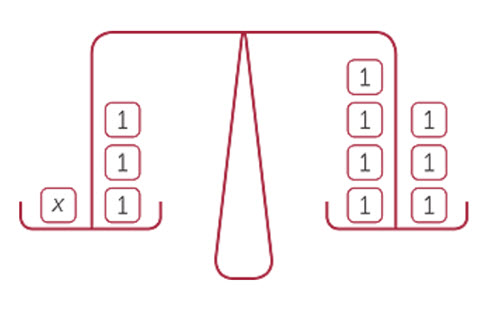Go to website

# Linear equations

This module from the AMSI 'The Improving Mathematics Education in Schools (TIMES) project' is for teachers who wish to consolidate their content knowledge on the topic of solving linear equations and using identities. There are sections that provide history and context for the topic, as well as exercises and examples of how to present and explain the concepts to students.

Year level(s) Year 7, Year 8
Purpose Content knowledge
Keywords linear equation, solve, identity, explicit teaching

## Curriculum alignment

Strand and focus Algebra
Topics Linear relationships
AC: Mathematics (V9.0) content descriptions
AC9M7A03
Solve one-variable linear equations with natural number solutions; verify the solution by substitution

AC9M8A02
Graph linear relations on the Cartesian plane using digital tools where appropriate; solve linear equations and one-variable inequalities using graphical and algebraic techniques; verify solutions by substitution

Numeracy progression Number patterns and algebraic thinking (P7)